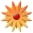# Mathematical Modeling & Inverse Variations

Page Views: 591

Email This Lesson Plan to Me
Email Address:
Subscribe to Newsletter?
Log in to rate this plan!
Overall Rating:(5.0 stars, 1 ratings)

 Keywords: Math Modeling, Data Collection, Visualization, Real World Data, Algebra Subject(s): Algebra, Geometry Grades 9 through 12 NETS-S Standard: Creativity and InnovationCommunication and CollaborationResearch and Information FluencyCritical Thinking, Problem Solving, and Decision MakingTechnology Operations and ConceptsView Full Text of Standards School: Mary Help Christians Academy, N Haledon, NJ Planned By: Edward Zambrano Original Author: Edward Zambrano, N Haledon
Students need to physically collect real data in order to understand the relationship that exists between the independent and dependent variables in an inverse variation. The exploration has students use a mirror, measuring tape, paper tape, and graphing calculator to collect, plot, and analyze data and then create a mathematical model to fit the data and make predictions. Students draw a diagram to reflect the collection process and note the motion of steps taken to connect the derived model and the proportionality that exists from the similar triangles formed. The visualization is key in comprehending the relationship whereby as one value increases the other decreases according to the proportion. The graphing calculator allows students to test models and test conjectures. Students need to study mathematics like science labs where they construct diagrams, make and test observations, and create math models. In this lesson, the students will read, interpret, and complete the exploration. The teacher will facilitate by asking leading questions and focusing attention on the data collection and the problem situation diagram which supports the mathematical model needed. Students will make predictions and then be able to test their values by returning to the collection process. Questions like, "How were you moving when the value was recorded ?" will support the inverse variation relationship. Many 'What If?' questions can be asked and answered during this lab.
 Comments We have limited access to graphing calculators because students are responsible for individual purchases. It is not possible for all students to make the individual purchase. These students need daily practice with the technology to be competitive on other assessments like SAT, ACT, AP examinations. Cross-Curriculum Ideas I work with our physics teacher who also teaches engineering and robotics as we incorporate STEAM objectives. Follow-Up Many data collection explorations - linear, quadratic, exponential, and trigonometric - will be incorporated as each unit is investigated to help students visualize, conjecture, and create appropriate mathematical models in an attempt to de-emphasize lecturing and facilitate exploration. Materials: Graphing, Batteries, High, Worksheets, Student Resources, Cause and Effect Other Items: 1 TI 84 Graphing Calculator Bundle , \$3400.00 each, total of \$3400.001 Communicators, \$600.00 each, total of \$600.00